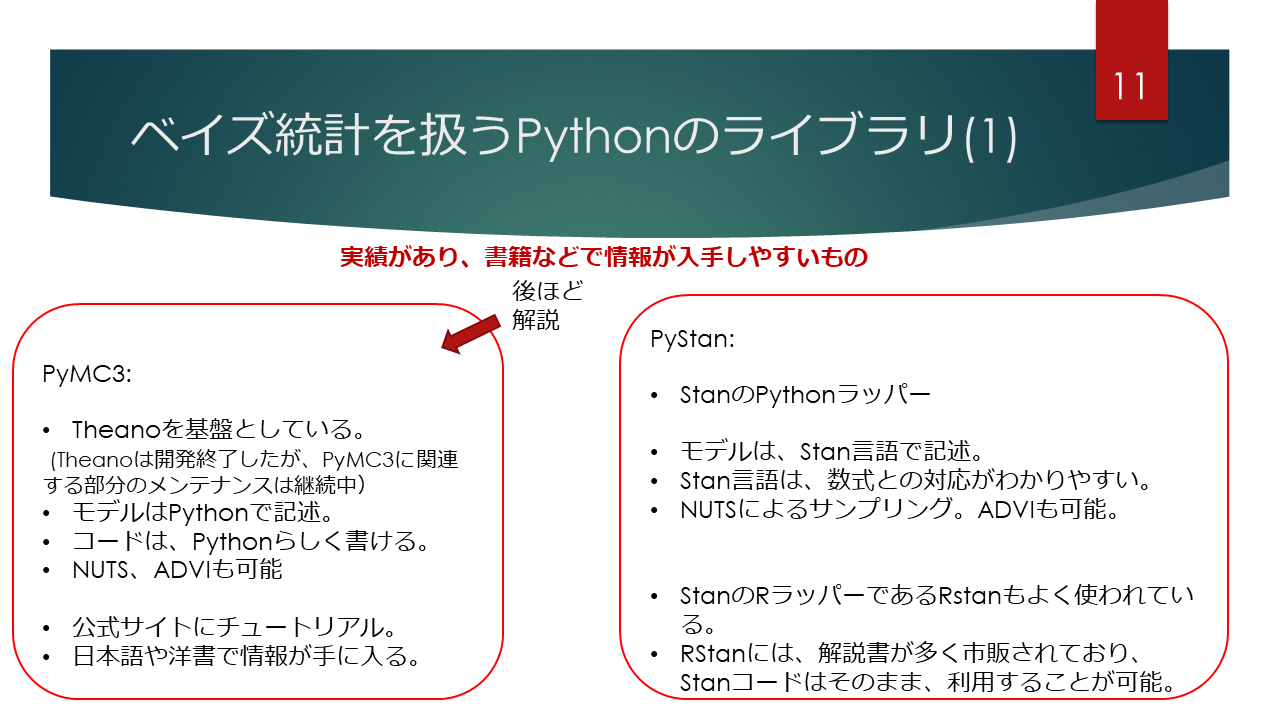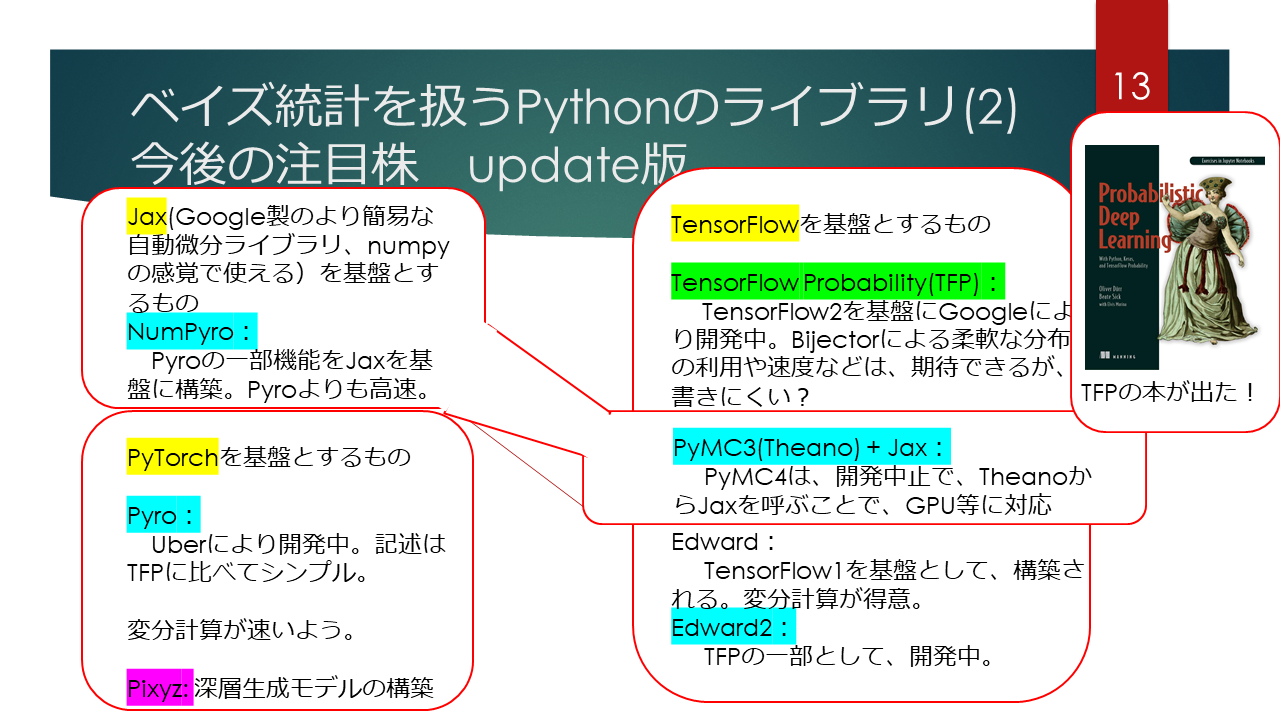# Hannari Python At the LT meeting in December, I made a presentation on "Python and Bayesian statistics".

This article is the 24th day article of Hannari Python Advent Calendar 2020 --Qiita. The day before, @ masayuki14 said, "I started Kaggle, so I'll do it little by little so that I can compete in the competition a year later." Nice to meet you, I'm Watanabe, @HW_a_pythonista, who is active on Twitter id. I am a regular of Hannari Python and made a presentation on Python and Bayesian statistics at the Hannari Python LT meeting in December, so I would like to summarize what kind of announcement I made on this page. (The above announcement is a reduction of what was announced at Pycon mini Hiroshima 2020 and includes some information updates.)

# What are Bayesian statistics?

Bayesian statistics is a statistic that reflects the observation data actually obtained from your prior probability (predicted probability) that you have before making an observation, and makes the best guess at that time. It is the method of. As for the method of machine learning, Bayesian statistics and Bayesian modeling are some kind of intermediates between the models with few parameters that are often used in statistics and the machine learning models that require tuning including many hyperparameters. You may think that. (In a rough way, I think there is a bad word)

# Why Bayes?

To explain why Bayes is, I would like to first look back on the recent situation surrounding AI and Python. The recent boom in AI and machine learning has been driven by methods such as deep neural networks that use large amounts of data. Here, Python has attracted attention and has become very popular. Python came into the limelight as deep learning libraries such as TensorFlow, Chainer, PyTorch, Theano, and MXnet were all recommended to be used from Python. In addition, the library of machine learning, such as Scikit-Learn and Numpy, which is not limited to deep learning, and the library of numerical calculation, which is the basis of it, also supported the popularity. Recently, parameter tuning called AutoTuner has been developed automatically, and at PyconJP2020, MLOps and the response speed of machine learning web applications were announced. Should data scientists and machine learning engineers only consider these issues in the future?

## Problems when using machine learning in the field

1. Interpretability In a Web system such as a shopping site, the Web system itself automatically returns a response. Therefore, even if the model is difficult to interpret, it is sufficient to adopt a model with good performance. However, in other practical applications, people are always involved in the system during operation. In that case, it is easy for people to not move just because the learning model as a black box performed better than before.

2. Amount of data For data with correct answer labels, it is possible to obtain a large amount of purchase data and which site to visit, and then to which site a large amount of history data can be obtained. However, in reality, there are not many problems that can obtain such a large amount of labeled data. In such a case, the machine learning method with many internal parameters makes it difficult to determine the parameters, and even if the parameters are determined, overfitting or performance difference from the simpler model is not possible. It will be difficult.

3. It is possible to reflect the nature of noise contained in the data in the learning model. If the data is derived from a physical measuring instrument, the error of that measuring instrument is naturally included. When performing regression, a Gaussian distribution is assumed, but this does not necessarily represent the nature of the instrument.

4. True generalization performance (which cannot be captured by cross-validation, etc.) In normal machine learning, the performance of the learning model is measured using methods such as cross-validation, and the optimum model is considered. However, in practical applications, a good model may be obtained by simply performing random cross-validation. This is when the number of data points is extremely small, the data points used for training and the data points used for testing, and the data points obtained in actual operation are far apart, or they belong to different clusters. Can happen to.

## Significance of Bayesian statistics and Bayesian modeling

One way to deal with these problems is to use Bayesian statistics and Bayesian modeling. Bayesian statistics can handle error information as a distribution, and by carefully incorporating the relationships behind the data, such as by introducing a hierarchical model, it is possible to deal with small amounts of data and bias.

# Introducing a library of Bayesian statistics that can be used in Python

## Those that have already been used a lot1. PyMC3 As the name suggests, PyMC is the third stage of the library that performs MC (Monte Carlo calculation) from Python, and was developed with the library Theano, which specializes in automatic differentiation, as the back end. Many sample codes are introduced on the official website, and many books are published, so learning is relatively easy. You can also use it from Google colabratory, and you can easily install it using conda or pip.

2. PyStan It is a Python wrapper of Stan, which is a typical software used for Bayesian modeling. Stan uses its own Stan language to describe models, but it has a grammar that makes it easy to deal with mathematical formulas. There are few books on PyStan, but many books on RStan have been published, so if you make the data reading and preprocessing part, you can make it work with the same Stan code and you can learn.

## Future attention stocks

In the future, I would like to write a library of Bayesian statistics that can be used from Python. The ones listed here are expected to handle large computational learning models to some extent. (I think that GPU speedup is also expected.)1. Based on Jax Jax is a relatively recent library of automatic differentiation made by Google. It is more popular than TensorFlow and PyTorch because it is a latecomer and easy to use like Numpy. , Numpyro based on that Jax. Variational calculation? It's fast, and PyMC4 has been developed based on TensorFlow Probability, which will be described later, but instead, the development has been changed to use Jax via Theano as the back end of PyMC3. It can be expected that the code assets already written in PyMC3 will be utilized as they are.

2. Based on PyTorch PyTorch has adopted define by run at a relatively early stage (although it is a latecomer to Chainer) such as deep learning, so the number of adoption exceeds TensorFlow, especially in academic fields. Pyro is a library based on PyTorch. The developer of Pyro is that Uber research department, and its reliability is expected because it is a library in which corporate resources are invested.

3. Based on TensorFlow and TensorFlow Probability TensorFlow is a library of tensor calculation developed by Google, and it has become the most popular as a deep learning framework, overtaking Caffe, Theano, etc. As mentioned above, PyTorch has been catching up with PyTorch recently, but in the commercial systems including machine learning currently in operation, the number used is still not large (most?). Is it? TensorFlow Probability is a library of stochastic programming based on TensorFlow. Since it is a stochastic programming library based on TensorFlow, it seems that it mainly sells high speed and detailed customization, but I think that it is a little difficult to write code compared to other libraries. However, an English book called "Probabilistic Deep Learning: With Python, Keras and TensorFlow Probability" has been released, so I think it would be a good idea to use this as a foothold to tackle issues such as deep learning + Bayes. think.

# Example of Bayesian modeling using PyMC3

We have introduced an example of modeling by linear regression when outliers are included, and an example of linear regression that introduces a hierarchical model for several populations. The former can be said to be an example that carefully reflects the knowledge about the error of data points, and the latter can be said to be an appropriate example by incorporating the information of the data of other groups even when the data for one group is small. It is an example of building a learning model.

# Summary

At the Hannari Python LT meeting in December 2020, we conducted an LT on "Python and Bayesian statistics". There are many libraries in Python that deal with Bayesian statistics. By performing Bayesian statistics and Bayesian modeling, it is possible to make a small turn compared to normal machine learning and obtain a solution that can reach the itchy place. Let's use it positively for everyone.